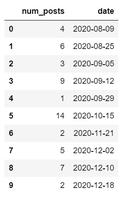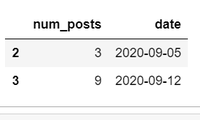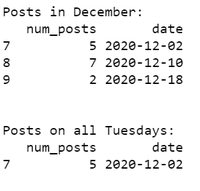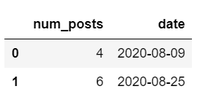Related Articles
How to Filter DataFrame Rows Based on the Date in Pandas?
• Last Updated : 11 Dec, 2020

Different regions follow different date conventions (YYYY-MM-DD, YYYY-DD-MM, DD/MM/YY, etc.).  It is difficult to work with such strings in the data. Pandas to_datetime() function allows converting the date and time in string format to datetime64. This datatype helps extract features of date and time ranging from ‘year’ to ‘microseconds’.

To filter rows based on dates, first format the dates in the DataFrame to datetime64 type. Then use the DataFrame.loc[] and DataFrame.query[] function from the Pandas package to specify a filter condition. As a result, acquire the subset of data, that is, the filtered DataFrame. Let’s see some examples of the same.

We will use a sample DataFrame consisting of the number of posts on a specific day. Convert the date in the sample data to datetime64 type as follows.

## Python

 `# Import Pandas package``import` `pandas as pd`` ` `# Create a sample dataframe``df ``=` `pd.DataFrame({``'num_posts'``: [``4``, ``6``, ``3``, ``9``, ``1``, ``14``, ``2``, ``5``, ``7``, ``2``],``                   ``'date'` `: [``'2020-08-09'``, ``'2020-08-25'``, ``'2020-09-05'``, ``                            ``'2020-09-12'``, ``'2020-09-29'``, ``'2020-10-15'``, ``                            ``'2020-11-21'``, ``'2020-12-02'``, ``'2020-12-10'``, ``                            ``'2020-12-18'``]})`` ` `# Convert the date to datetime64``df[``'date'``] ``=` `pd.to_datetime(df[``'date'``], ``format``=``'%Y-%m-%d'``)`` ` `# Display dataframe``df`Example 1:

Filter data based on dates using DataFrame.loc[] function, the loc[] function is used to access a group of rows and columns of a DataFrame through labels or a boolean array. In this example, the conditional statement in loc[] returns a boolean array with True value if row satisfies condition (date is in between 1st and 15th September) and False value otherwise. Then the loc[] function returns only those rows having True values.

## Python3

 `# Import Pandas package``import` `pandas as pd`` ` `# Create a sample dataframe``df ``=` `pd.DataFrame({``'num_posts'``: [``4``, ``6``, ``3``, ``9``, ``1``, ``14``, ``2``, ``5``, ``7``, ``2``],``                   ``'date'``: [``'2020-08-09'``, ``'2020-08-25'``, ``                            ``'2020-09-05'``, ``'2020-09-12'``, ``                            ``'2020-09-29'``, ``'2020-10-15'``,``                            ``'2020-11-21'``, ``'2020-12-02'``, ``                            ``'2020-12-10'``, ``'2020-12-18'``]})`` ` `# Convert the date to datetime64``df[``'date'``] ``=` `pd.to_datetime(df[``'date'``], ``format``=``'%Y-%m-%d'``)`` ` `# Filter data between two dates``filtered_df ``=` `df.loc[(df[``'date'``] >``=` `'2020-09-01'``)``                     ``& (df[``'date'``] < ``'2020-09-15'``)]``# Display``filtered_df`

Output:Example 2:

Use the DateTimeIndex(dt) to access separate date time attributes such as year, month, day, weekday, hours, minutes, seconds, microseconds etc. as a condition in loc[] function as follows.

Note: The date values should be in datetime64 format.

## Python3

 `# Import Pandas package``import` `pandas as pd`` ` `# Create a sample dataframe``df ``=` `pd.DataFrame({``'num_posts'``: [``4``, ``6``, ``3``, ``9``, ``1``, ``14``, ``2``, ``5``, ``7``, ``2``],``                   ``'date'``: [``'2020-08-09'``, ``'2020-08-25'``,``                            ``'2020-09-05'``, ``'2020-09-12'``,``                            ``'2020-09-29'``, ``'2020-10-15'``,``                            ``'2020-11-21'``, ``'2020-12-02'``,``                            ``'2020-12-10'``, ``'2020-12-18'``]})`` ` `# Convert the date to datetime64``df[``'date'``] ``=` `pd.to_datetime(df[``'date'``], ``format``=``'%Y-%m-%d'``)`` ` `# Filter data between two dates``filtered_df ``=` `df.loc[(df[``'date'``] >``=` `'2020-09-01'``)``                     ``& (df[``'date'``] < ``'2020-09-15'``)]`` ` `# Display``print``(``"\nPosts in December:"``)``print``(filtered_df)`` ` `# Filter data for specific weekday (tuesday)``filtered_df ``=` `df.loc[df[``'date'``].dt.weekday ``=``=` `2``]`` ` `# Display``print``(``"\nPosts on all Tuesdays:"``)``print``(filtered_df)`

Output:Example 3:

Filter data based on dates using DataFrame.query() function, The query() function filters a Pandas DataFrame and selects rows by specifying a condition within quotes. As shown below, the condition inside query() is to select the data with dates in the month of August (range of dates is specified). The columns of the DataFrame are placed in the query namespace by default so the date column can be accessed without indexing (simply specify column name).

## Python3

 `# Import Pandas package``import` `pandas as pd`` ` `# Create a sample dataframe``df ``=` `pd.DataFrame({``'num_posts'``: [``4``, ``6``, ``3``, ``9``, ``1``, ``14``, ``2``, ``5``, ``7``, ``2``],``                   ``'date'``: [``'2020-08-09'``, ``'2020-08-25'``,``                            ``'2020-09-05'``, ``'2020-09-12'``,``                            ``'2020-09-29'``, ``'2020-10-15'``,``                            ``'2020-11-21'``, ``'2020-12-02'``,``                            ``'2020-12-10'``, ``'2020-12-18'``]})`` ` `# Convert the date to datetime64``df[``'date'``] ``=` `pd.to_datetime(df[``'date'``], ``format``=``'%Y-%m-%d'``)`` ` `# Filter data between two dates``filtered_df ``=` `df.query("date >``=` `'2020-08-01'` `\``                       ``and` `date < ``'2020-09-01'``")`` ` `# Display``filtered_df`

Output:Attention geek! Strengthen your foundations with the Python Programming Foundation Course and learn the basics.

To begin with, your interview preparations Enhance your Data Structures concepts with the Python DS Course.

My Personal Notes arrow_drop_up